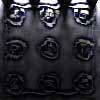#### You may also like### Fixing the Odds

You have two bags, four red balls and four white balls. You must put all the balls in the bags although you are allowed to have one bag empty. How should you distribute the balls between the two bags so as to make the probability of choosing a red ball as small as possible and what will the probability be in that case?### Scratch Cards

To win on a scratch card you have to uncover three numbers that add up to more than fifteen. What is the probability of winning a prize?### Escalator

At Holborn underground station there is a very long escalator. Two people are in a hurry and so climb the escalator as it is moving upwards, thus adding their speed to that of the moving steps. ... How many steps are there on the escalator?

# Guessing the Graph

##### Age 14 to 16 Challenge Level:

When analysing scientific data, we often need to suggest a curve to fit a set of points, and deduce the equation of the curve.

In this set of cards, there are three data sets. Print and cut out one of the sets, shuffle the cards, and then choose a few points.

By plotting the points you are given, can you sketch a curve representing the relationship between the two variables?

The data were recorded in three sets, A, B and C, beginning at three interesting points in the experiment, so you may wish to investigate each set separately. Alternatively, you can choose to investigate the combined datasets.

Can you come up with a possible relationship using only a few pieces of data?

Start with a few data points. Plot them on a set of axes and try to fit a curve to the data.

Select some more values - do they fit your curve? Draw a new curve in a different colour if you want to change your original "best fit".

Once you are satisfied that your curve represents the data well, try working out an approximate equation for your curve, perhaps using a spreadsheet.

What sort of experiment do you think generated the data?
What sort of scientific questions might be answered by using the graph?
Based on your idea of what the experiment was, why do you think the maximum points of curves B and C are lower than the maximum point of curve A?